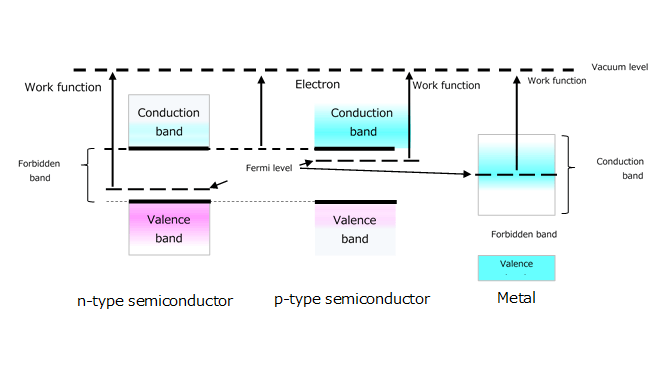# 2. Metal-semiconductor junction

In the previous section, we have discussed the diffusion potential across a pn junction.
You now understand that the Fermi level is the reference energy level for the pn junction.
In the case of a metal-semiconductor junction, a metal and a semiconductor are joined together so that their Fermi levels line up with no external bias. Although the Fermi level is used as a reference point for the diffusion potential (barrier potential) of a pn junction, for a metal-semiconductor junction the work function is used as a reference point. The characteristics of the metal-semiconductor junction depend on whether the work function of the metal is greater or less than that of the semiconductor as described later.
These are some terms used here:

• Electron affinity: Generally, the electron affinity of an atom or a molecule is defined as the amount of energy released when an electron is attached to a neutral atom or molecule. The electron affinity of a semiconductor is a difference between the lowest energy level in the conduction band and the vacuum level. The electron affinity of a metal is equal to its work function.
• Fermi level: The Fermi level is the energy level that has a 50% probability of being occupied by an electron according to the Fermi distribution
• Work function: The work function is the amount of energy needed to remove a free electron from a molecule. It is equal to a difference between the Fermi and vacuum levels.
• Vacuum level: The vacuum level is the energy level of a charged particle (e.g., an electron) when it is in a vacuum with zero kinetic energy.Figure 2-1 Relationship between Fermi level and work function in semiconductors and metals

When an n-type semiconductor with a work function of Φn and a metal with a work function of Φm are joined together, the characteristics of the metal-semiconductor junction depend on the difference between Φn and Φm. A Schottky junction is formed when Φm > Φn whereas an ohmic junction is formed when Φm < Φn. The work function of a semiconductor is the Fermi level minus the vacuum level.

## Related information

A new window will open